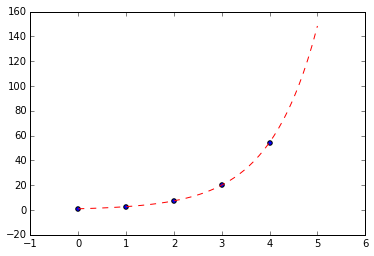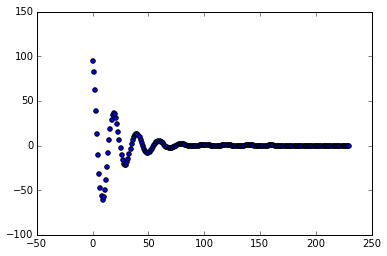# Runge-Kutta Method¶

Solve $y'=y$, $y(0)=1$ using RK4 method.

In :
import numpy as np
import math
import matplotlib.pyplot as plt
%matplotlib inline
step = 1
x = np.arange(0, 5, step)
y = np.zeros(x.size)

def derivative(x, y):
return y

y = 1
for i in range(x.size-1):
k1 = derivative(x[i], y[i])
k2 = derivative(x[i]+step/2.0, y[i]+0.5*k1*step)
k3 = derivative(x[i]+step/2.0, y[i]+0.5*k2*step)
k4 = derivative(x[i]+step, y[i]+k3*step)
y[i+1] = y[i]+(step/6.0)*(k1+2*k2+2*k3+k4)

plt.scatter(x,y)

x = np.linspace(0, 5, 50)
y = math.e**x
plt.plot(x, y, 'r--')

Out:
[<matplotlib.lines.Line2D at 0x1085316d0>]# Case Study: Spring-mass Damper System¶

The system diagram:A block is suspended freely using a spring. The mass of the block is M, the spring constant is K, and the damper coefficient be b. If we measure displacement from the static equilibrium position we need not consider gravitational force as it is balanced by tension in the spring at equilibrium.

The equation of motion is $Ma = F_{s}+F_{d}$ where $F_{s}$ is the restoring force due to spring. $F_{d}$ is the damping force due to the damper. $a$ is the acceleration.

The restoring force in the spring is given by $F_{s}= -Kx$ as the restoring force is proportional to displacement it is negative as it opposes the motion. The damping force in the damper is given by $F_{d}=-bv$ as damping force is directly proportional to velocity and also opposes motion.

Therefore the equation of motion is $Ma=-Kx-bv$.

Since $a=\frac{dv}{dt}$ and $v=\frac{dx}{dt}$ we get

$M\frac{dv}{dt}=-Kx-bv$ and $\frac{dx}{dt}=v$.

In :
# source: http://gafferongames.com/game-physics/integration-basics/
import numpy as np
import math
import matplotlib.pyplot as plt
%matplotlib inline

x = 100
v = 0
t = 0
dt = 0.1

def acceleration(x, v, t):
k = 10
b = 1
return -k*x - b*v

def evaluate1(x, v, t):
dx = v
dv = acceleration(x, v, t)
return (dx, dv);

def evaluate2(x, v, t, dt, derivatives):
dx, dv = derivatives
x += dx*dt
v += dv*dt
dx = v
dv = acceleration(x, v, t+dt)
return (dx, dv);

def integrate(t, dt):
global x
global v
k1 = evaluate1(x, v, t);
k2 = evaluate2(x, v, t, dt*0.5, k1)
k3 = evaluate2(x, v, t, dt*0.5, k2)
k4 = evaluate2(x, v, t, dt, k3)
k1_dx, k1_dv = k1
k2_dx, k2_dv = k2
k3_dx, k3_dv = k3
k4_dx, k4_dv = k4
dxdt = (1/6.0)*(k1_dx+2*(k2_dx+k3_dx)+k4_dx)
dvdt = (1/6.0)*(k1_dv+2*(k2_dv+k3_dv)+k4_dv)
x += dxdt*dt
v += dvdt*dt

xData = []
vData = []
while abs(x) > 0.001 or abs(v) > 0.001:
integrate(t, dt)
t += dt
#print "x=",x," v=",v
#print x
xData.append(x)

plt.scatter(np.arange(len(xData)),xData)

Out:
<matplotlib.collections.PathCollection at 0x1091259d0>In [ ]: